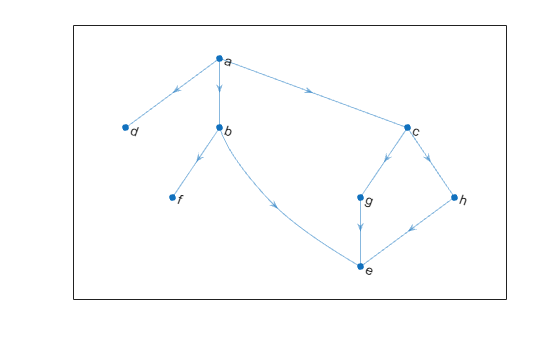# successors

Node successors

## Syntax

``sucIDs = successors(G,nodeID)``

## Description

example

````sucIDs = successors(G,nodeID)` returns the successor nodes of the node in directed graph `G` specified by `nodeID`. The successor nodes form directed edges with `nodeID` as the source node, and `sucIDs` as the target nodes.```

## Examples

collapse all

Create and plot a directed graph and determine the successor nodes of node `'a'`.

```s = [1 1 1 2 2 3 3 7 8]; t = [2 3 4 5 6 7 8 5 5]; names = {'a' 'b' 'c' 'd' 'e' 'f' 'g' 'h'}; G = digraph(s,t,[],names); plot(G)````sucIDs = successors(G,'a')`
```sucIDs = 3x1 cell {'b'} {'c'} {'d'} ```

## Input Arguments

collapse all

Input graph, specified as a `digraph` object. Use `digraph` to create a directed graph object.

Example: `G = digraph([1 2],[2 3])`

Node identifier, specified as one of the values in this table.

ValueExample
Scalar node index`1`
Character vector node name`'A'`
String scalar node name`"A"`

Example: `sucIDs = successors(G,3)` finds the successor nodes of node 3.

## Output Arguments

collapse all

Successor node IDs, returned as a node indices or node names.

• If `nodeID` is a numeric node index, then `sucIDs` contains numeric node indices.

• If `nodeID` is a node name, then `sucIDs` contains node names.

## Tips

• For multigraphs with multiple edges between the same two nodes, the output of `outedges` can be longer than that of `successors`, since there can be multiple outgoing edges to some of the successors.